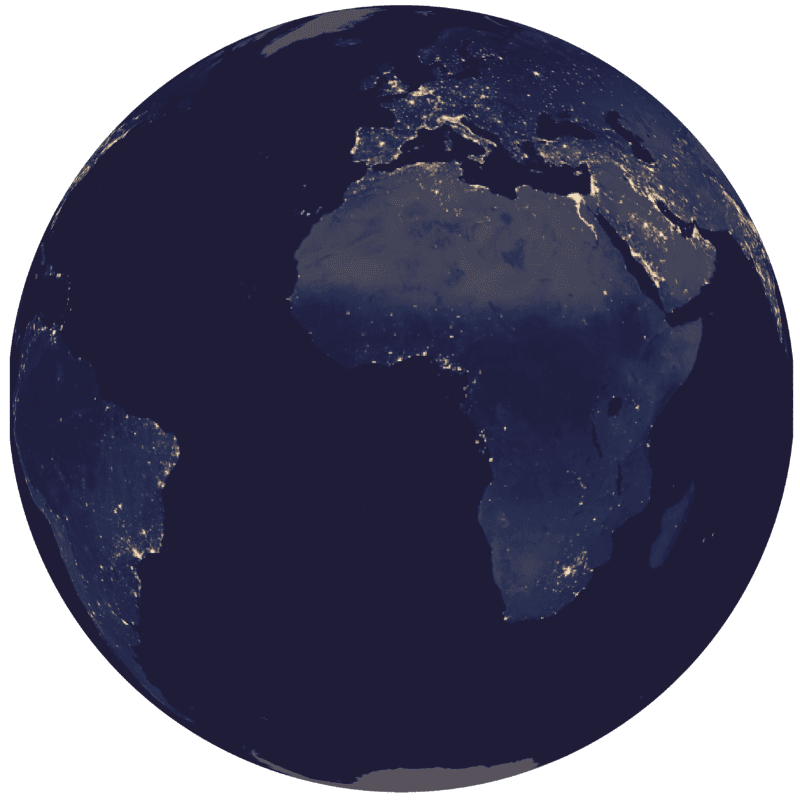# Spinning Globes With RIt has been a long held dream of mine to create a spinning globe using nothing but R (I wish I was joking, but I’m not). Thanks to the brilliant mapmate package created by Matt Leonawicz and shed loads of computing power, today that dream became a reality. The globe below took 19 hours and 30 processors to produce from a relatively low resolution NASA black marble data, and so I accept R is not the best software to be using for this – but it’s amazing that you can do this in R at all!The code I used to do this is posted below. Under the hood the ggplot2 package is used for the plotting, so the first few data prep steps are taking the raster and converting it into a format that works with that. For information about installing the required packages and some other examples see the mapmate vignette here – there’s a lot more to it than the below. The black marble dataset is from here.

#Load in the packages
library(raster)
library(mapmate)
library(dplyr)
library(geosphere)
library(data.table)
library("parallel")
library(purrr)
library(RColorBrewer)
library(classInt)
marble<-raster("BlackMarble_2016_3km_geo.tif")
#Simplify the raster - to test this out I would set the value at 50+
marble<- aggregate(marble, 10)
#Extact the values and coordinates from the raster grid - this is what is passed onto ggplot2.
marble.pts<-rasterToPoints(marble, spatial=T)
marble.pts@data <- data.frame(marble.pts@data, long=coordinates(marble.pts)[,1],lat=coordinates(marble.pts)[,2])
names(marble.pts@data)<- c("z","lon","lat")
#Convert to the tidyverse, required for mappmate.
marble.dat<-as_data_frame(marble.pts@data)
#We aren't plotting the image colours - rather a series of rectangles to be coloured by the pixel value. The range of colours needs to be specified. For this I have extraced the main colours from NASA's image and approximately aligned them to their corresponding values. This is seen the colour palette below that gets fed into the map.
pal<-colorRampPalette(c("#0b0c1a","#1e1c37","#202144","#2b3355","#7f6e61","#d0b695","#efd7af", "#fefbe6"), bias=2.75)
n<-30
#some more magic here - see the vignette I link to above.
marble.frame <- map(1:n, ~mutate(marble.dat, frameID = .x))
rng <- range(marble.dat\$z, na.rm=TRUE)
file <- "3D_rotating_simp"
id<- "frameID"
#OK - here goes! You need the parallel package up and running - mc.cores specifies how many processors to use. You can see I used 30 but this can obviously be less.
mclapply(marble.frame, save_map,z.name="z", id=id, lon=0, lat=0, n.period=30, n.frames=n, col=pal(5000), type="maptiles", file=file, z.range=rng,png.args = list(width = 30,
height = 30, res = 300, bg = "transparent", units="cm"),rotation.axis = 0,mc.cores=30)

James Cheshire